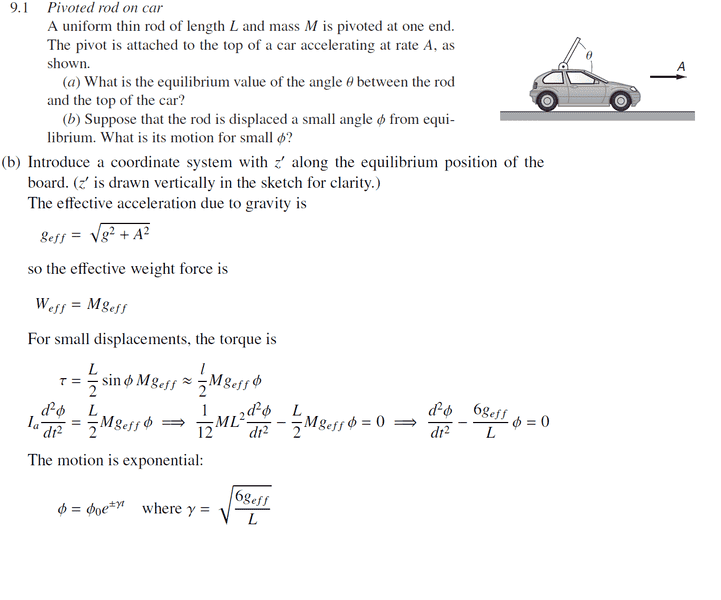# Linear fictitious force problem

## Homework Statement## The Attempt at a Solution

I understand the solution of the problem, but I want to know why don't my approach work.

I didn't change the frame of reference.
I use the regular method of solving a torque problem.
(mgsinθ - mAcosθ)(L/2) = mL2/3 θ''
which is approximately equal to
gθ-A = 2/3 L θ''
by solving differential equations, I got a frequency of Root (3/2 * g/L) , why am I wrong? Thank you (Also, isn't the moment of inertia of a rod 1/3 * mL^2 with respect to the rod?)

## Answers and Replies

Charles Link
Homework Helper
Gold Member
2020 Award
Yes, the moment of inertia of the rod about the pivot point is ## I_a=\int\limits_{0}^{L} \rho \, r^2 \, dr =(\frac{1}{3})ML^2 ##. ## \\ ## That will get you the correct answer. ## \\ ## ( It's only ## (\frac{1}{12})ML^2 ## if you rotate it about its center).